Hello and Welcome!!
Take this simple QUIZ and see how much you need an AVO-AVA course1 / 5
50%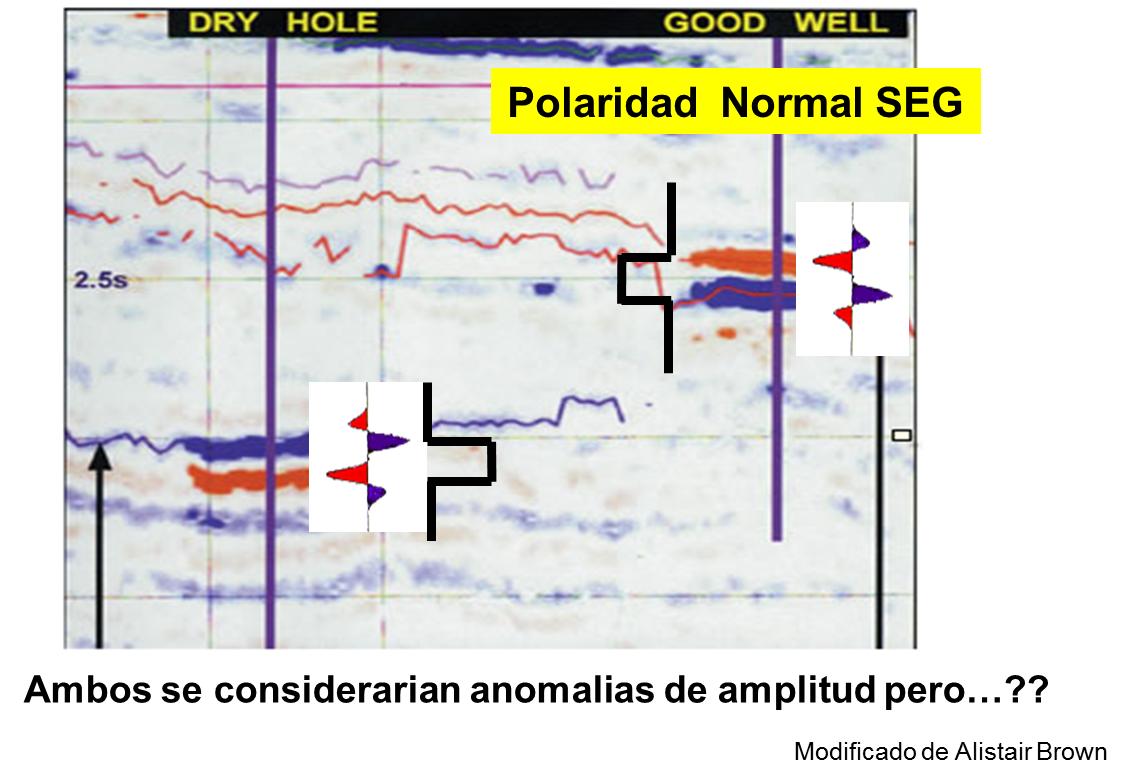Stacked amplitudes are a good representation of the Normal Incidence reflectivity.
1 / 5
50%

### Whta is the name of the equations that explain the process of energy partition at the surface between two elastic media, with different elastic properties ?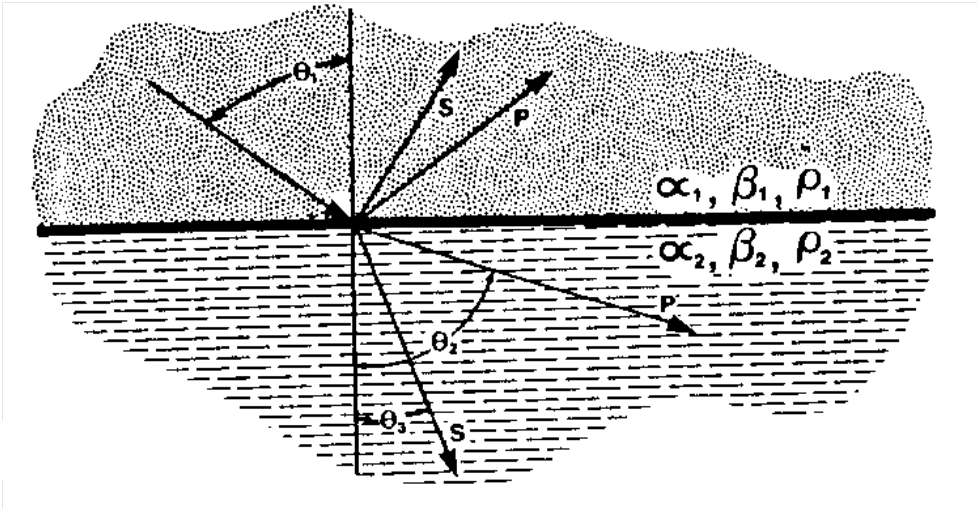Thanks for taking the QUIZ. It is only a fine sample but it may help you decide how mucho you know about the topic.Estas ecuaciones son importantes en física, pero no son las correctas.1 / 5
50%

### The critical parameter for converting de original recording data from offsets to angles of incidence is: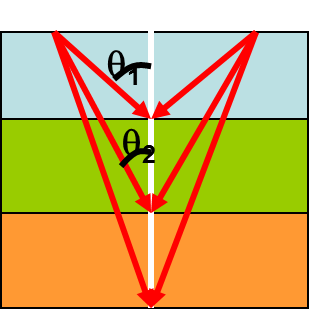Nope... it's a geometric conversion where depth is involved... and since our data is recorded in time the conversion is simple... we need the RMS velocity1 / 5
50%

### The results from AVA proccesing represent: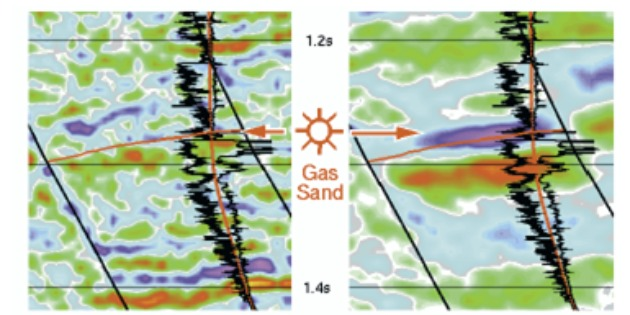Incorrect... the analyzed process is a reflection process, and as such the results are elastic reflectivities.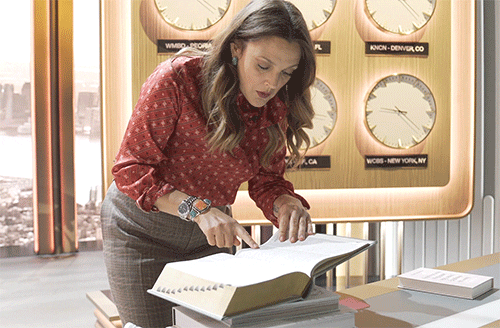1 / 5
50%

### Which of the approximations to the Zoeppritz equations is the simplest:They are all approximations, and therefore simpler than Zoeppritz, but of these there is one that stands out for its simplicity in terms a and b of a linear equation y=a+bx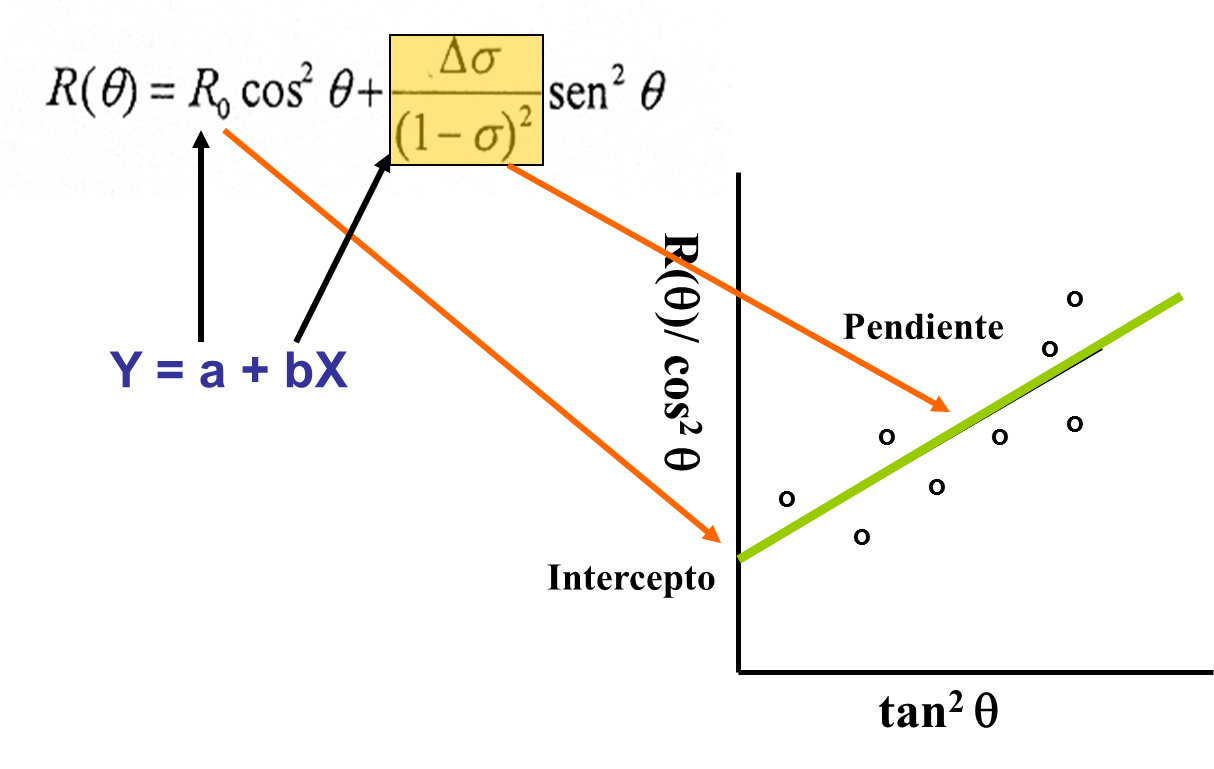Incorrect... normally there is some kind of AVA effects which makes that the normal incidence values differ from the stacked values.